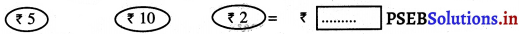# PSEB 3rd Class Maths Solutions Chapter 5 Money (Currency)

Punjab State Board PSEB 3rd Class Maths Book Solutions Chapter 5 Money (Currency) Textbook Exercise Questions and Answers.

## PSEB Solutions for Class 3 Maths Chapter 5 Money (Currency)

PAGE 120:

Do you remember?

Question 1.
Add the amount of coins :

(a)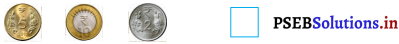Solution.
17

(b)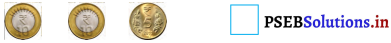Solution.
25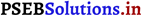Question 2.
Convert the given amount into coins.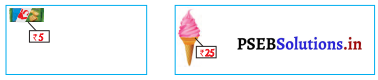Solution.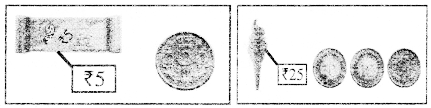PAGE 125:
Let’s do:

Question 1.
Mark > or < between two coins: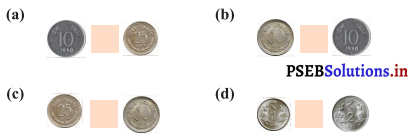Solution.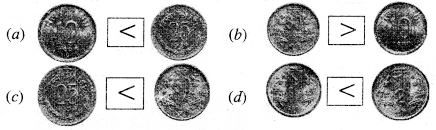PAGE 126:
Let’s do:

Prepare coins: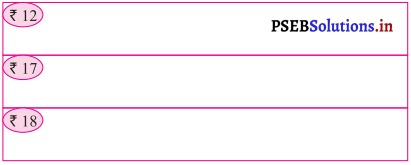Solution.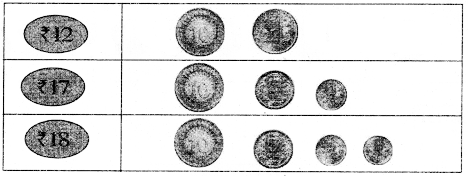PAGE 128:
Let’s do:

Make currency flotes or coins for the given article: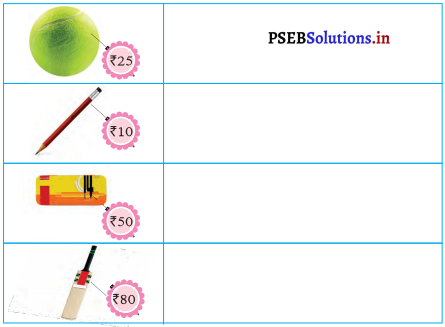Solution.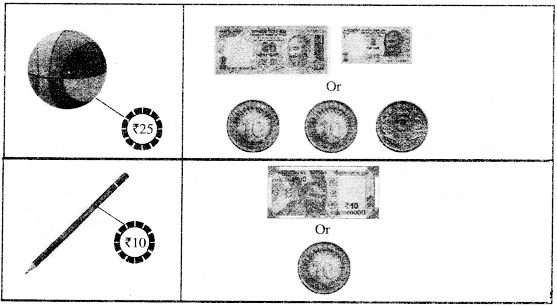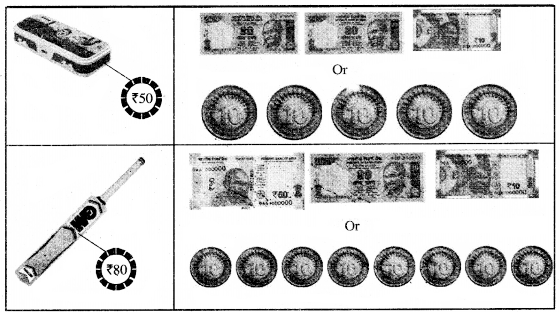PAGE 131:
Let’s do:

Question 1.
Kamal bought balloons for ₹ 40. Lovepreet bought balloons for ₹ 33. How much amount did they spend together to buy these balloons ?Solution.
Kamal spent on balloons for = ₹ 40
Lovepreet spent on.balloons for = ₹ 33
Total amount of money spent = ₹ 40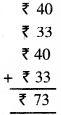Therefore, amount they spent on balloon = ₹ 73

Question 2.
Honey had ₹ 36 in his money bank. He put ₹ 23 more into it. How much amount is in Honey’s money bank now?Solution.
Amount in Honey’s money bank = ₹ 36 9
More amount Honey put in money bank = ₹ 23
Total amount of money in Honey’s money bank = ₹ 59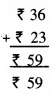Thus amount in Honey’s money bank = ₹ 59PAGE 132:
Let’s do:

Subtraction of Money:

Question 1.
Meena had ₹ 85. She bought a geometry box for ₹ 35. How much money is left with her ?
Solution.
Amount of money Meena has = ₹ 85
Cost of geometry box = ₹ 35
Amount of money left with Meena = ₹ 50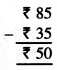Therefore, ₹ 50 left with Meena.

Question 2.
Jagveer had ₹ 77. He bought a chocolate for ₹ 15. How much money is left with him ?
Solution.
Cost of a chocolate = ₹ 15
Amount of money left with Jagveer = ₹ 62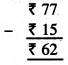Therefore, ₹ 62 left with Jagveer.Question 3.
Nazia had ₹ 66. She bought a pen for ₹ 12. How much money does she have now ?
Solution.
Amount of money Nazia had = ₹ 66
Cost of a pen = ₹ 12
Amount of money, left with Nazia = ₹ 54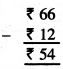Therefore Nazia has ₹ 54 now.

PAGE 133:
Let’s Learn:

Bill amount of cash memo: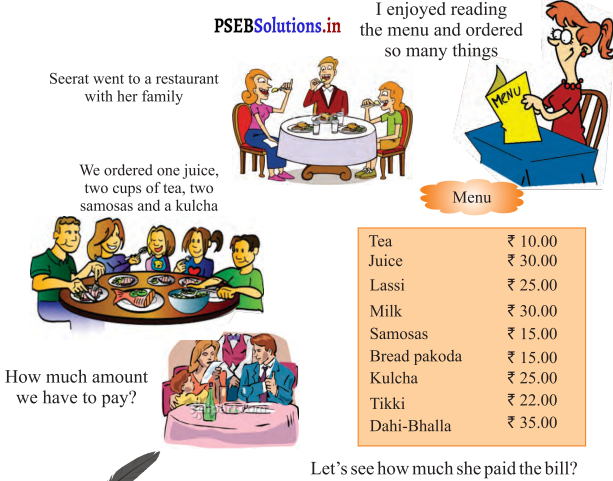Let’s see how much she paid the bill ?

Let’s do: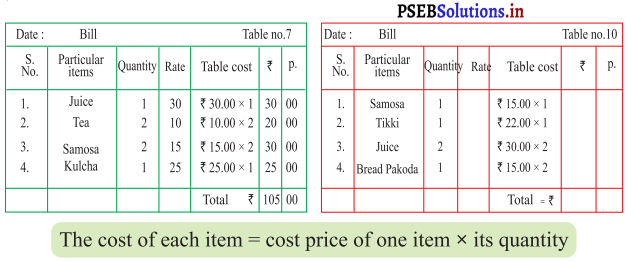Solution.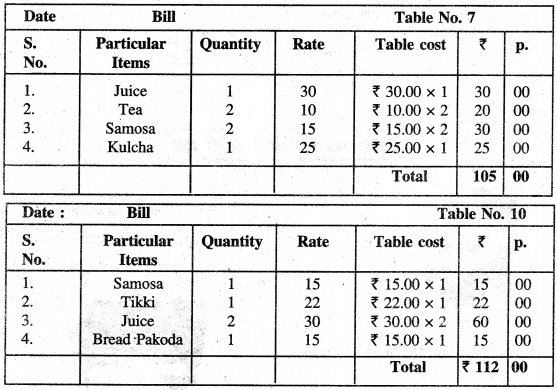PAGE 134:

Worksheet:

Question 1.
Convert rupees into paise :
(a) ₹ 9 = ________
Solution.
900 paise

(b) ₹ 6 = ________
Solution.
600 paise

(c) ₹ 2 = ________
Solution.
200 paise

d) ₹ 8 = ________
Solution.
800 paiseQuestion 2.Solution.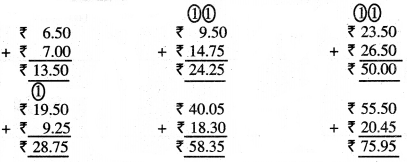Question 3.
Sutract: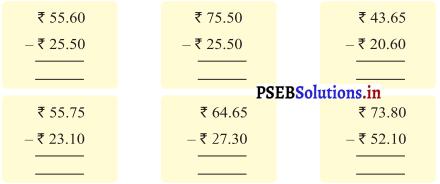Solution.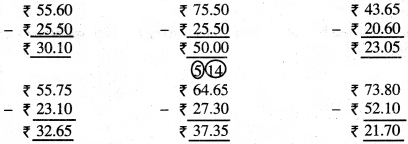Question 4.
Count and write :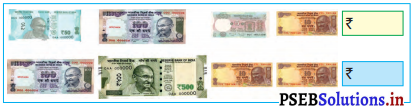Solution.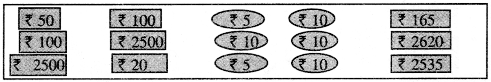Multiple Choice Questions:

Question 1.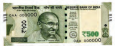= ________ paise.
(a) 100
(b) 200
(c) 400
(d) 500
Solution.
(d) 500

Question 2.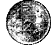= ________ paise
(a) 100
(b) 200
(c) 300
(d) 400
Solution.
(b) 200Question 3.